# The diameter and heights of lunar craters

### Abstract

In this experiment you will use images of the Moon (taken with the School's telescope) to identify impact craters on the lunar surface. You will then go on to measure the diameters of the craters and their heights. Finally, you will compare the results you have obtained with published values.

### Introduction

Before the advent of spaceflight our knowledge of the Moon was limited to what could be gleaned from meticulous observations of its surface using gound-based drawings and photographs. Eventually, space missions, such as Apollo, revolutionized our understanding of the lunar topography and geology (actually selenology).

Here you will use images taken with our charge-couple device (CCD) detector attached to our 10" telescope to make measurements of the diameters and heights of craters.

### Section 1: The data

The data consist of a series of 8 images that were taken on 13 March 2003. The images were taken along the terminator of the Moon, which is the line that separates the day and night hemispheres. You will see that substantial amounts of the images are a uniform white, and that some show streaks of white in the vertical direction. This is where the CCD images are "saturated"; the Moon is very bright and some of the pixels in the camera have become full of charge, which may have then leaked into surrounded pixels. You should ignore regions of the images that are saturated, as they do not contain useful data.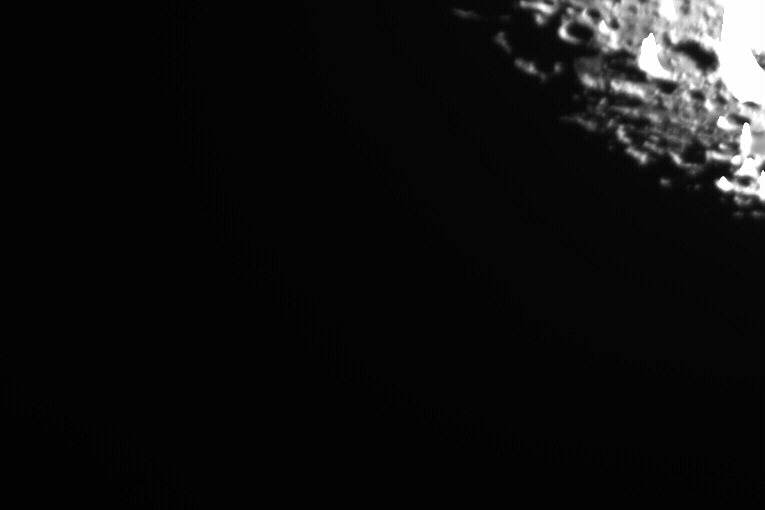Image 1: 2003 March 13 19:25 UT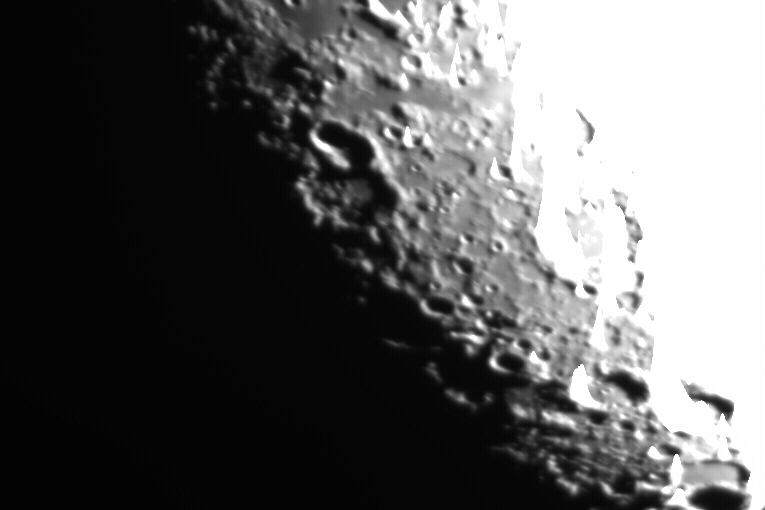Image 2: 2003 March 13 19:27 UT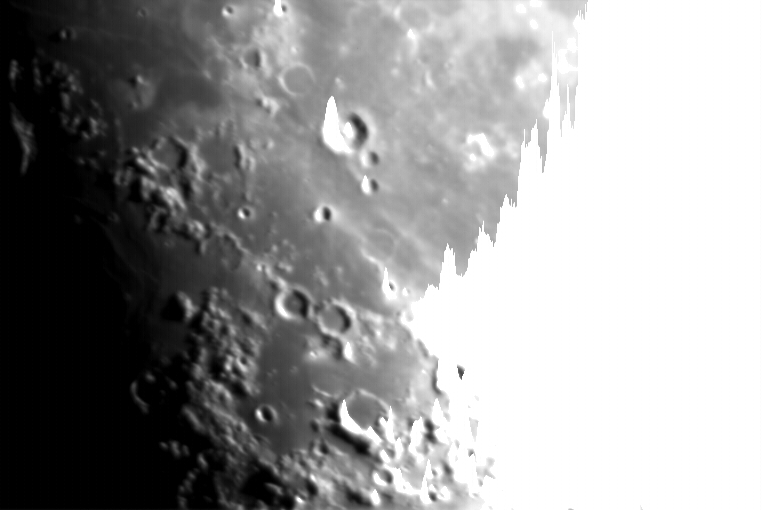Image 3: 2003 March 13 19:28 UT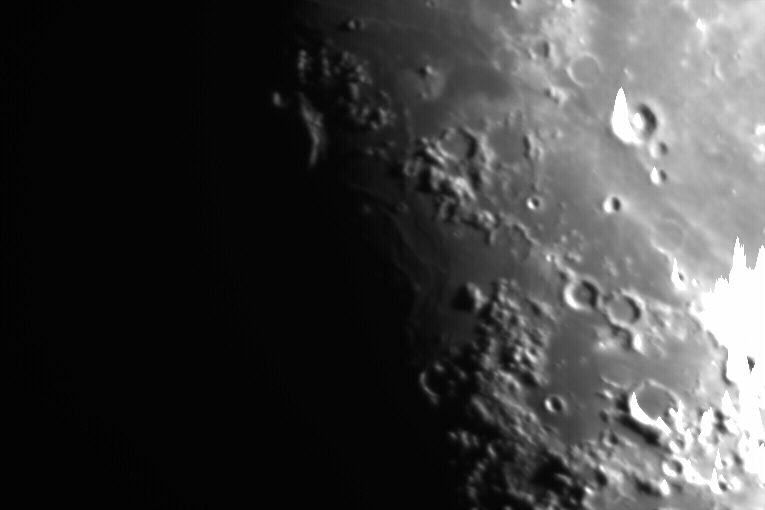Image 4: 2003 March 13 19:30 UT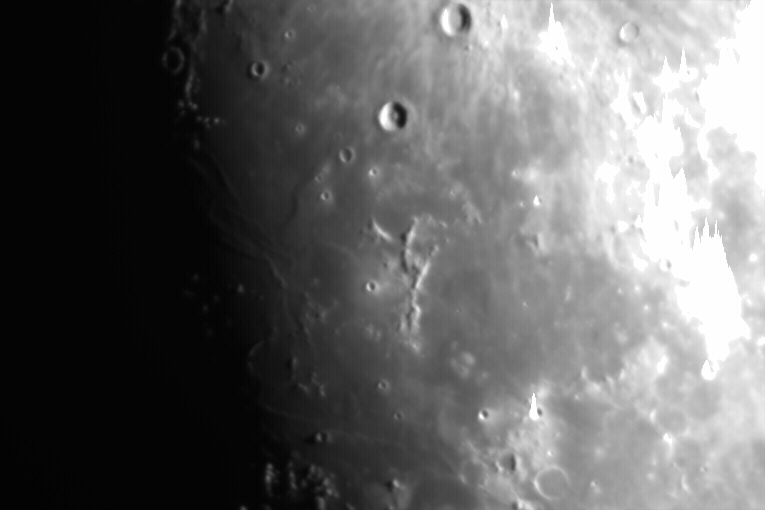Image 5: 2003 March 13 19:31 UT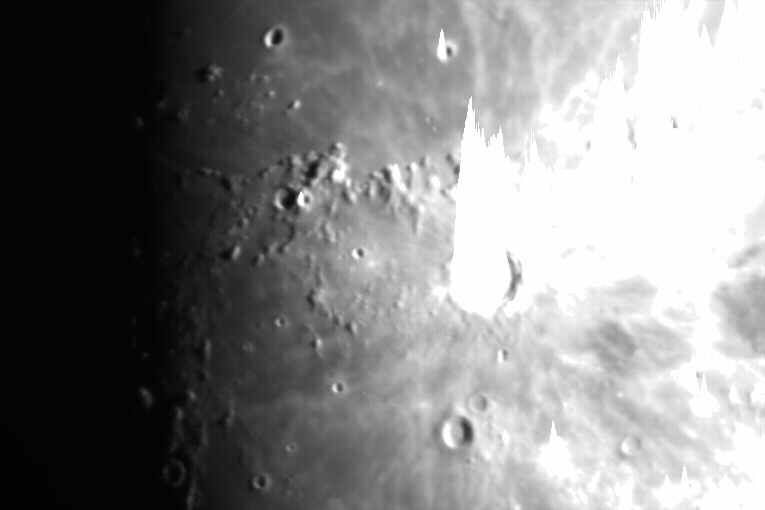Image 6: 2003 March 13 19:32 UT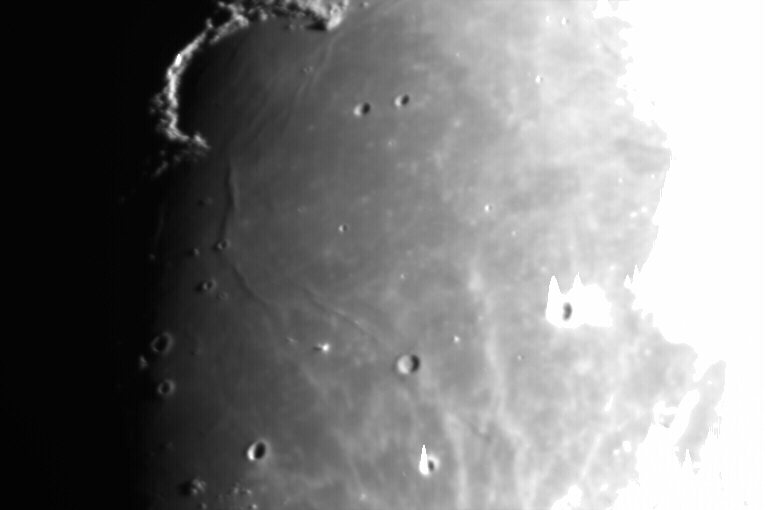Image 7: 2003 March 13 19:32 UT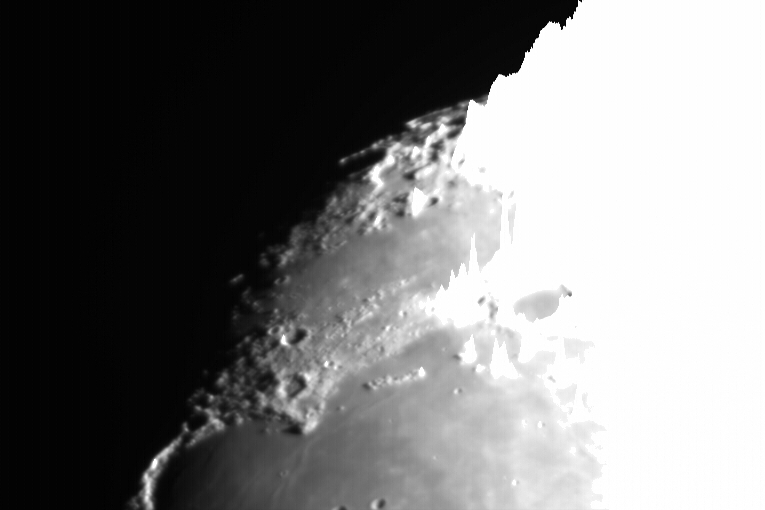Image 8: 2003 March 13 19:33 UT

The images in the above table are links to jpeg format copies of the original images and you should download these to your user space. On the Macs in the lab you will find an application called ImageJ (in the Applications folder) which is suitable for viewing these images.

The lunar surface comprises dark, flat regions known as maria (singular mare, latin for sea), brighter regions which are highlands, and of course the ubiquitous impact craters. The craters are especially conspicuous near the terminator, where their shadows are longest. Many craters contain interior planes that are as flat as the maria, and mountainous walls that define their edges. The largest crater on the near side of the Moon is Clavius (diameter 235km), and the curvature of the lunar surface is such that if you were standing in the middle of this crater you would not be able to see its walls (which are 6.6 km high).

Use the online lunar atlas to identify the main features on each image. Print out a copy of each of the images and annotate them with the names of the features. Hint: as a starting point compare this atlas image with images number 7 and 8.

### Section 2: Measuring the diameters of craters

Identify five craters that you wish to measure. These should show a measurable shadow (so look near the terminator) and should appear in this list of craters. Make sure you write down the latitude and longitude of the craters. The latitude and longitude are in the selenographic coordinate system, where the zero-point is in the centre of the disc facing Earth.

Question: Why did you select your particular craters?

You now need to measure the diameter of the crater in pixels. In ImageJ the x and y co-ordinates of the pixel under the cursor are shown in the ImageJ window. Due to projection effects (foreshortening) the crater will appear as an ellipse; always measure the longest axis. Estimate your error on this value. We know that the pixel scale of the image is 0.73 arcseconds per pixel (remember that an arcsecond is 1/60 of an arcminute which is in turn 1/60 of a degree). You can now calculate the apparent angular diameter of the crater. In order to determine the physical diameter of the crater we need to know the lunar distance, which (since its orbit about the Earth is eccentric) varies with time. Use this lunar ephemeris program and the UT time of the image that you are interested in to find the lunar distance at the time of the observation (note down all the information given, you'll need it later). Now convert the lunar distance (given in Earth radii) to metres, and hence calculate the diameter of your crater in kilometres and its associated error. Compare this with the value in the database, and comment on the accuracy of your measurements. Hint: you may find the small angle approximation useful.

### Section 3: The heights of craters

The principle behind measuring the heights of lunar craters is quite simple: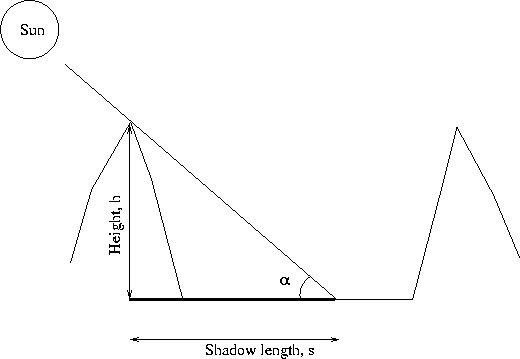For a given crater the Sun is at an elevation α. The quantity measured is the shadow length s. It is then trivial to work out the height h of the crater, measured from the crater floor to the top of the crater rim.

There is a subtly here though. The shadow length observed will only be the same as the measured length s if the crater is viewed directly from above. Otherwise the shadow is foreshortened, and the projected length we measure is shorter than the true length. We need to compensate for this factor, so divide the apparent length of the shadow by the cosine of the crater's selenographic longitude to get the true length.

Question: Why doesn't the foreshortening change with latitude along the terminator?

We now need to calculate α, which is the Sun's elevation viewed from the crater at the time the image was taken. This requires some spherical trigonometry: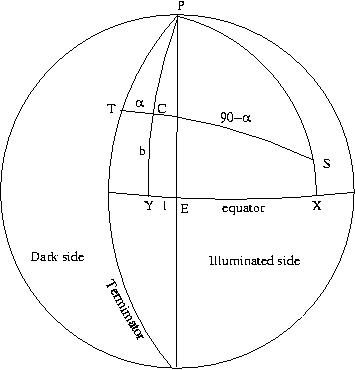The above figure shows the lunar disc, with the crater marked at C. The sub-earth point which defines the zero-point of selenographic coordinates is marked E, while the pole is denoted P. The terminator, which divides the illumated part of the disc from the dark part is also shown. If you were standing on the lunar surface at S at the time of the observation the Sun would be directly overhead, and this is called the sub-solar point. Obviously the angular distance from S, through the crater C, to the terminator at T, is 90 degrees. (If you were standing on the terminator then the Sun would be on the horizon). We are interested in the angle of Sun above the horizon at C, which we have called α.

The angle CY is the selenographic latitude of the crater, while angle EY is its longitude. Similarly, angle SX is the selenographic latitude of the sub-solar point, and EX is its longitude (this quantities are given by the ephemeris program). We can now apply the cosine equation for a spherical triangle to get:

cos(CS)=cos(PC)cos(PS)+sin(PC)sin(PS)cos(CPS)

here CPS is the vertex angle, and is the difference in longitudes between the sub-solar point and the crater (be careful about signs here). Solve this equation for CS, and hence α (remember since your crater is close to the terminator you expect this angle to be small). You are now in a position to calculate your crater height, and its associated error.

Compare your crater heights with those in the literature - try this page for depths of craters (in feet, so convert to metres). Comment on the accuracy of your measurements.

Question: How could you improve the accuracy of the experiment?